# 1st PUC Chemistry Question Bank Chapter 4 Chemical Bonding and Molecular Structure

## Karnataka 1st PUC Chemistry Question Bank Chapter 4 Chemical Bonding and Molecular Structure

### 1st PUC Chemistry Chemical Bonding and Molecular Structure One Mark Questions and Answers

Question 1.
What is chemical bonding ?
Chemical bonding is the attractive force that holds or binds constituents were atoms, ions or molecules together in a substance.

Question 2.
Why do atoms combine ?
Atoms have tendency to attain the stable electronic configuration octet structure of the nearest noble gas in the periodic table.

Question 3.
Name the types of chemical bonds.
(i) Ionic bond
(ii) Covalent bond
(iii) Co-ordinate bond
(iv) Hydrogen bond
(v) Metallic bond

Question 4.
Noble gas does not form compounds. Why ?
Noble gas has stable electronic configuration in the valence shell ns2np6 (octet structure) helium has two electrons only.

Question 5.
What is octet rule ?
The tendency of atoms of elements to attain octet structure of the nearest noble gas during formation of a chemical bond is known as octet rule.Question 6.
What is meant by octet structure ?
The atoms having 8 electrons in the outer most orbit or having valence electronic configuration ns2n p6.

Question 7.
Why are rare gases are mono atomic ?
Rare gases process octet structure i.e., 8-electrons in the outer most orbit. They have no tendency to react.

Question 8.
Give one molecule that does not obey octet rule.
Hydrogen molecule.

Question 9.
Define bond length.
It is defined as average distance between the centers of nuclei of the two bonded atoms in a molecule.

Question 10.
Define polarization.
The tendency of the anion to get distorted or polarized by the cations is called polarization or polarisability.Question 11.
Between AICl3 and AIF3 which is covalent ?
AICl3.

Question 12.
Name the type of bonds present in ice ?
Covalent bonds and intermolecular hydrogen bonds.

Question 13.
What is the type of hybridization exhibited by Boron in Boron trifluoride ?
sp2.

Question 14.
What is H-C-H bond angle in methane ?
109° 28′.

Question 15.
How many sigma bonds are present in ethane ?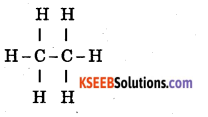There are 7a bonds in ethane.Question 16.
What angles are associated with the following orbitals ? sp, sp2 and sp3.
sp = 180°
sp2 = 120° ‘
sp3 = 109°28′

Question 17.
What is London forces ?
It is a weak force of attraction between non-polar molecules like O2 or N2 (i.e, between like atoms).

Question 18.
Mention the type of bond formed between the atoms having same electronegativity.
Covalent bond.

Question 19.
Mention the type of bond formed between the atoms having different electronegativity.
Ionic bond.

Question 20.
Who received noble price for the work on chemical bonds.
Linus pauling. .

Question 21.
What is an ionic bond ?
An ionic bond is the electrostatic force of attraction between oppositely charged ions. Or A chemical bond formed by complete transfer of one or more electrons from outermost orbit of electropositive atom to outer most orbit of electronegative atom.

Question 22.
Give an example for ionic crystal ?
Sodium chloride crystal, magnesium chloride.

Question 23.
Among HF, HC1, HBr and III which has highest ionic character ?
HFQuestion 24.
What do you mean by electrovalence ?
Number of ions lost (gained) by atoms during the formation of ionic bond.

Question 25.
Give any two factors which favours ionic bond.
Low ionization energy for metals and high electron affinity of non-metals.

Question 26.
Define lattice energy.
The energy associated when are mole of ionic crystal is formed from its gaseous ions.

Question 27.
What you mean by Born-IIaber cycle ?
It is cycle of operation needed for the formation of an ionic crystalline solid from its gaseous ions as element.

Question 28.
What is meant by covalent bond ?
A chemical bond formed by the sharing up of one or more electrons between the atoms.

Question 29.
What is covalency ?
Number of electrons shared between the atoms during covalent bond formation.

Question 30.
What is the covalency of CH4 ?
4

Question 31.
Who proposed concept of covalent bond ?
In 1916, G.N. Lewin

Question 32.
What is the maximum covalency of chlorine ?
+7Question 33.
Who proposed valency bond theory ?
In 1927, Heitlen and London.

Question 34.
Draw a diagram to show orbital overlap to form a σ bond.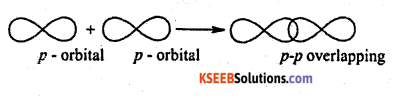Question 35.
Draw a diagram to show the orbital overlap to form π-bond.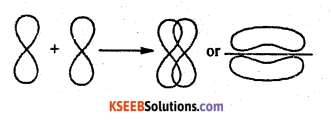Question 36.
Draw a diagram for sigma p-p overlapping.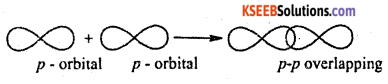Question 37.
What is a sigma bond ?
Sigma bond is a bond formed by the overlapping of atomic orbital along the axis.

Question 38.
What is a pi bond ?
A bond formed between two atoms by the sideways overlapping of two half filled p-orbitals is called as a pi bond.Question 39.
How many sigma and pi bonds are present in an acetylene molecule ?
H – C = C – H Four sigma bonds and two pi bonds.

Question 40.
How many pairs of electrons are involved in bond formation in case of nitrogen molecule.
Three pairs.

Question 41.
What is hybridization ?
It is a process where two or more atomic orbital of comparable energy of valency shell of an atom mix to form the same number of new orbital of same energy.

Question 42.
Mention the overlapping present in
(a) H2
(b) Cl2
(c) HCl
(a) s – s
(b) p – p
(c) s – pQuestion 43.
How many electrons are shared in a double bond between two atoms ?
4 (four)

Question 44.
Which type of hybridization is called (a) tetrahedral (b>trigonal ?
(a) sp3, b) sp2.

Question 45.
Give example for two linear molecule.
Ethyne and CO2.

Question 46.
Who proposed VSEPR theory ?
In 1940 by Sidgwick and Powell.

Question 47.
What is the structure of ammonia & water molecule ?
Pyramidal and Bent structure respectively.

Question 48.
Mention the band angle in Ammonia & H2O,
107° and 104° respectively.

Question 49.
What is meant by non (homo) polar covalent bond ?
In a covalent bond if the pair of electrons shared equally (symmetrically) between the atoms called non-polar covalent bond.Question 50.
What is meant by hetero (polar) covalent bond ?
In a covalent bond if the pair of electrons are shared unequally (asymmetrically) between the atoms are called polar covalent bond.

Question 51.
Give example for polar and non-polar molecule.
Polar HCl, H2O, NH3, Non-polar H2, O2, Cl2.

Question 52.
Write the relationship between dipole movement and charge.
µ = exd (where µ = dipole moment, e = charge d = distance)

Question 53.
Define Hydration energy.
The energy released when an ionic solid is dissolved is water resulting is polarization of water molecules to form hydrated ions, called hydration energy.Question 54.
What is dipole moment?
If is the product of the distances of charge (positive or negative) centres and distance between the centre.

Question 55.
Which among the following has zero dipole moment BF3, µH3, H2O ?
BF3

Question 56.
What is coordinate bond ?
In a chemical bonding both the electrons for sharing between atoms are contributed (donated) by one atom only.Question 57.
Give one example for the molecule conducting covalent & coordinate bond.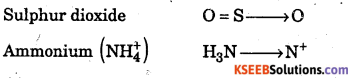Question 58.
Give one example of a compound containing ionic, covalent and coordinate

Question 59.
Which type of bond formed between NH3 and BF3 ?
Co-ordinate bond.

Question 60.
Who proposed the concept of co-ordinate bond ?
G. A. Perkin in 1921.

Question 61.
What is hydrogen bond ?
The attractive force which binds hydrogen atom of one molecule with electronegative atom present is the same or other molecule is called hydrogen bond.Question 62.
Name two types of hydrogen bonding.
Inter molecular and Intra molecular hydrogen bond.

Question 63.
At what temperature water has maximum density ?
4°C

Question 64.
How are formic acid molecular are associated ?
Formic acid molecules are associated with inter molecular hydrogen bonding

Question 65.
Name the property by which the dissolution of an ionic solid in water is possible.
Hydrogen bonding.

Question 66.
What is Vender Waal’s force ?
It is a weak inter atomic or inter molecular force of attractions existing in substances without formation of any chemical bond.### 1st PUC Chemistry Chemical Bonding and Molecular Structure Give Reasons Questions and Answers

Question 1.
Why “Ionic bond is formed between alkali metals and halogens” ?
Because Alkali metals have low first ionization energy while halogens have high electron affinity.

Question 2.
Ionic solids do not conduct electricity.
In ionic solids, ions occupy certain fixed positions in the crystal and do not migrate.

Question 3.
Ionic compounds are insoluble in ether.
Ionic compounds does not ionize in ether i.e. ether cannot separate the cations and anions of ionic solids.

Question 4.
7π bond is weaker than σ bond.
In sigma band the overlapping is maximum where as in pi band the overlapping is minimum-

Question 5.
Dipole moment of CO2 is zero.
In the linear molecule O = C = O, the dipole due to oxygen from both sides are equal & opposite. As a result dipole moment is zero.Question 6.
Water molecule has bent structure even it undergoes sp3 hybridization.
Due to the repulsion between the two lone pair of electrons in water molecule, the structure become bent.

Question 7.
Boiling point of water is very high.
Due to the presence of intermolecular hydrogen bonding in water.

### 1st PUC Chemistry Chemical Bonding and Molecular Structure Two Marks Questions and Answers

Give Reason Question:

Question 1.
1. Give any two difference between σ and π bond.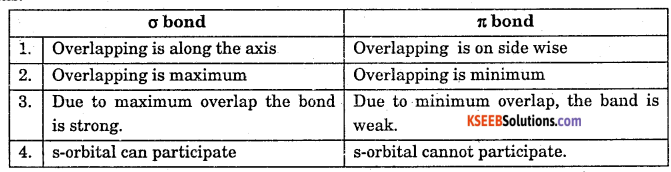Question 2.
Mention the π and π bond present in (1) N2 (2) O2 (3) C2H4 (4) C2H2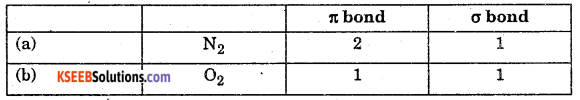Question 3.
Write any two conditions for (factors favouring) covalent bond.
1) Two atoms have half filled orbitals
2) Shared electrons have opposite spins.
3) Overlapping must be maximum.

Question 4.
Which type of overlapping result in the formation Of (i) sigma bond (ii) pi bond.
(i) Sigma bond-overlapping of orbital along the axis.
(ii) pi bond overlapping sideways of two p-orbital.

Question 5.
What is a Bond angle ? Give example.
It is defined as the “angle between the oribitals containing bonding electron pairs around the central atom in a molecule or in a complex ion”. It is expressed in degrees. Example : In CO2 the bond angle is 180°. So CO2 has linear shape.Question 6.
What is bond energy ? Give example.
It is defined as “the amount of energy required to break one mole of bonds of a particular type in the gaseous state.” It is also called bond dissociation enthalpy. Example : C – C bond enthalpy is 348 kJ mol’1.

Question 7.
What is bond order ? Give example.
It is the number of covalent bonds holding the atoms in the molecule.
Example : If the bond is formed by the sharing of two electron pairs, then the bond order is 2. O = O or C = C bond in alkenes.

Question 8.
Calculate the bond order of Helium.
Helium contains four electrons. Its electronic configuration is H2
Bond order = $$\frac{1}{2}$$ (nb -na)
nb = number of bonding electrons
na = number of anti bonding electrons
$$\frac{1}{2}$$(2-2) =0
So, He2. does not exist.

Question 9.
Explain Van-der-waals force with example.
It can be explain with 3 types.
(a) Dipole and Dipole interaction : Dipole – Dipole interaction exists between polar molecules like NH3, SO2, HC1… Greater the dipole interaction greater is Vander Waal’s force. Hence such molecules liquefied easily.
(b) Dipole & induced dipole interaction : A permanent dipole molecule can induce polarity in a non-polar molecular when comes near to each other. As a result between polar of non-polar molecule there exists force of attraction.
(c) Induced dipole & Induced dipole interaction. Non polar moleculas like, O2 and N2 having induced polarity on each other which is called Landon forces. When these two molecules comes near to each other the force increases.

Question 10.
Explain the term ‘Hydration of Ions”.
When ionic solid is dissolved in polar solvent like water, the cations & anions of ionic solid is attracted by oxygen and hydrogen of water respectively. As a result each ions surrounded by many number of water molecule to form hydrated ion. This exothermic process is called Hydration.

* Degree of hydration depends upon the charge density of an ion.
• Degree of hydration decreases as size of ion increases
Example : Li+ > Na+ > K+
• Ionic solid dissolves in water easily, if lattice energy is less than hydration
energy.

Question 11.
Give example for s – p overlapping.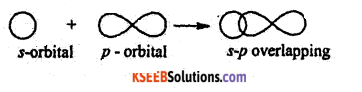Question 12.
Give example for p – p σ overlapping.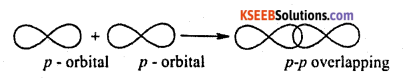Question 13.
Why do C show 4 valency ?
The ground state electronic configuration of C is 1s22s22p2. In exited state, one electron if 2s jumps to 2p, hence the electronic configuration 1s2 2s12p1x 2p1y 2p1z

Question 14.
Draw the sp3. hybridized C.
In sp3 hybridization from 1s22s12p1x 2p1y 2p1z the orbitals 2s22p1x 2p1y 2p1z hybridization to give sp3 hybridized C as follows.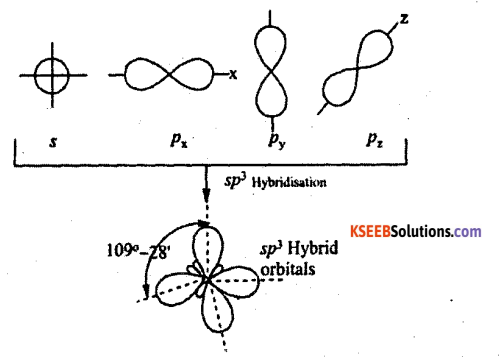Question 15.
Draw the sp2 hybridized C.
In sp2 hybridization, the 2s1,2p1x,2p1y orbtials hybridization where as 2pz is not involves in hybridization.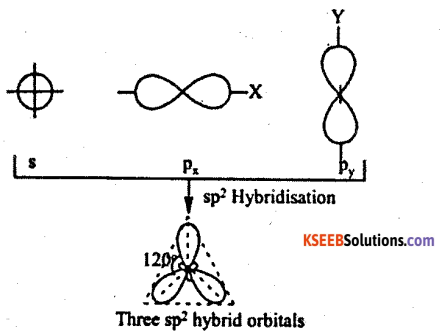Question 16.
Draw the sp hybridized C.
In sp hybridized C, the 2s1 &2p1x is hybridizes where as 2p1y and 2p1z is not.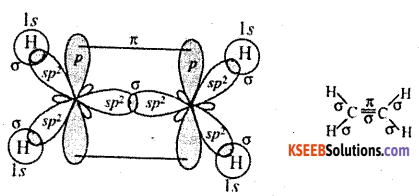Question 17.
What do you mean by lone pair of electron ?
The pair of electron which involves in hybridization but not involves in covalent bond formation (involves in co-ordinate bond formation).

Question 18.
Mention the condition favouring ionic bond formation.

1. Lower Ionisation energy of metals.
2. Higher Electron affinity of non – metal
3. Higher magnitude of lattice energy of bond
4. Higher electromagnativity difference between metal and non-metals.

Question 19.
Mention any two characteristics of ionic compounds.

1. They have high Melting point.
2. Many of it are solid in nature.
3. Does not conduct in solid state but good conductors in fused or aqueous state.
4. Soluble in polar solvent & insoluble in non-polar solvent.
5. Ionic bond is non-directional.
6. They do not exhibit Isomerism.Question 20.
Mention the factors favouring covalent bond.
In covalent molecule the combining atoms should have

1. High ionization energy
2. High electron affinity
3. Small or equal electronegative
4. Smaller atomic size
5. Contain unpaired electron with opposition spin
6. Attain octect structure.
7. Smaller intermolecular distance.

Question 21.
Mention the characteristic of covalent molecule.

1. They are generally gases or liquid or soft solid
2. Insoluble in polar solvent & soluble in non polar solvent.
3. They do not conduct.
4. They always exists in its molecular state but not in ionic state.
5. They have fixed direction in space.
6. They show isomerism.
7. They do not undergoes electrolysis hence called non-electrolytes.

Question 22.
How do Vander Waal’s force establishes ?
Electrons in non-polar molecule oscilates (vibrates). As a result the charges will be v conentrated in a region. Hence non-polar molecule becomes momentarily polarized. This polarized molecule shows induced (artificial) dipole moment which attracts the constituents together – results is Vander Wall’s force.Question 23.
What do you mean by Co-ordinate Bond ? Explain with example.
The bond formed by donation of electron from one of the constituents present in the molecule is called co-ordinate bond.
It is only directional bond & indicated by ” → ”
H3 → BF3 [Nitrogen donates pair of electrons to form bond] NH3BF3

Question 24.
HC1 form polar bond.
In HCl electron is not shared equally, since chlorine is more electronegative than hydrogen and the shared pair shifts more towards chlorine. As a result partially charged Hδ+ – Clδ- result polarity.

Question 25.
Ionic solid do not conduct.
In it the ions occupy certain fixed lattice positions in the crystals and do not move. Hence they do not conduct.

Question 26.
NH3 polar but BF3 non-polar.
Ammonia has dipole moment due to pyramidal structure but is BF3 the dipole moment is zero due to symmetrical planar structure.Question 27.
HF is liquid where as HCl, HBr…. Other hydrogen halide is liquid.
Since F is highly electronegative, name there exists powerful inter molecular hydrogen bonding in HF results in liquid state.

Question 28.
O-Nitrophenol is more volatile than p-Nitrophenol (Or) boling point of p- nitriphenol is greater than O-nitrophenol)
In O-nitrophenol there exists intra molecular hydrogen bonding within the molecule where as in p-nitrophenol there exists inter molecular hydrogen bonding between the molecule.

Question 29.
Covalent compounds have law melting point.
In covalent compound molecules are held together by weak intermolecular forces.

Question 30.
H2O is liquid whereas H2S is gas.
In H2O, hydrogen is combines with highly electronegative element and also forms inter molecular hydrogen bond. But S is less electronegative and in H2S there is no hydrogen bonding.

Question 31.
C – H bond is polar but CH4 is non polar.
Methane has symmetrical tetrahedral structure hence dipole moment is zero.
Therefore it is non polar.

Question 32.
Density of water is maximum at 4°C.
Along with covalent bond and hydrogen bond, H2O molecule exists in best form from tetrahydral from state by leaving vacant space. When heated from 0°C > 4°C the ‘ hydrogen bond brakes and H2O modecule comes closer. As a result density increases to maximum at 0°C. From 4°C above, due to heat examples takes place by braking the the destroy decreases.Question 33.
Water is polar in nature.
Even though sp3 hybridization is there is H2O but due to lone pair – lone pair replace
there exists.

Question 34.
Water has high boiling point.
Due to intermolecular hydrogen bonding, water has high boiling point.

Question 35.
Ice floats on water or ice is lighter than water.
Along with covalent bond & hydrogen bond, ice form of water exists in the tetrahydral shape from bent structure by leaving vacant space. Due to this vacant space is ice if floats in water.

Question 36.
CO2 and C2H2 (acetylene) are non-polar.
Because both have linear structure.

Question 37.
σ bond is stronger compare to π bond.
In a σ bond the overlapping is along the axis and is maximum extent. Where as π bond overlapping is sidewise and overlapping is minimum.Question 38.
Ethyl alcohol is an organic compound but still freely soluble in water explain.
The solubility of ethyl alcohol in water is due to the presence of intermolecular hydrogen bonding between the two.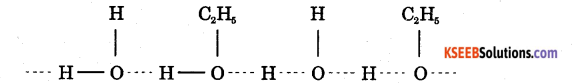Energy is released during the attraction this helps in the dissolution of alcohol in water.

Question 39.
Nitrogen and chlorine have the same electro negativity but only former forms hydrogen bonds. Explain.
Both these elements have the same elecro negativity (3.0). But the size of nitrogen . atom (atomic radius = 75pm) is smaller than that of chlorine (atomic radius = 99pm). As a result nitrogen atom can cause greater polarization of N – H bond than the Cl atom in Cl – H bond. Thus hydrogen bonding is present in H – H bonds (e.g., NH3) but not in a – H bonds (eg. HCl)

### 1st PUC Chemistry Chemical Bonding and Molecular Structure Three Marks Questions and Answers

Question 1.
Explain the formation of Ionic bond in NaCl.
Sodium has electronic configuration 1s2 2s2 2p6 3s1, when it looses one electron,
attains stability where as chlorine 1s22s22p63s23p5, it gain one electron to get stability.
∴ The electron lost by Na is gained by the chlorine forming ionic bond.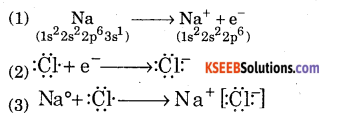Question 2.
Write the Barn Haber cycle for the formation of NaCl.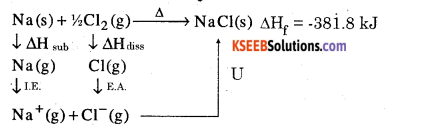When U = Lattice energy ; ∆HS – Enthalpy for sublimation, ∆Hi = Enthalpy of ionization, ∆Hd = enthalpy of dissociation, ∆HEa = Enthalpy for electron affinity.
The only one step for formation of NaCl from sodium and chlorine is associated with the energy called = ∆Hf = enthalpy of formation.
∆Hf = U + ∆HS + ∆Hi + ∆Hd + ∆HEa
∴ Lattice energy U = ∆Hf= [∆HS + ∆Hi + ∆Hd + ∆Hta ]Question 3.
Explain the steps involved in Born Haber cycle for the formation of NaCl.
Step 1 : Sublimation of metallic sodium of gaseous sodium atom with enthalpy of
sublimation = ∆HS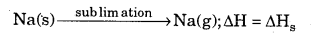Step – 2 : Dissociation of molecule of chlorine to chlorine atoms with enthalpy of dissociation = ∆Hd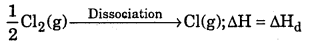Step – 3 : Ionization of gaseous sodium with enthalpy of ionization = ∆Hi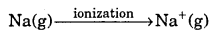Step – 4 : Addition of electron to gaseous chlorine atom with enthalpy of electron affinity = ∆HEa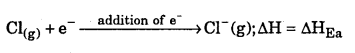Step – 5: Close packing of gaseous sodium ion and chloride ion to form lattice structure of NaCl, with lattice chloride ion to form lattice structure of NaCl, with
Lattice energy = U; NaCl+(g) + Cl(g) → NaCl∆; ∆H = U

Step – 6 : But sum of all the energies will be equal to the heat of formation of one mole of sodium chloride form its reluctant i.e., ∆Hf
Na(s) + 1/2Cl2(g) >NaCl(s);∆H = ∆Hf

Question 4.
Define hybridization ? Explain the hybridization in Methane molecule.
CH4 – Methane Molecule.
It is a process where two or more atomic orbital of comparable energy of valency shell of an atom mix to form the same number of new orbital of same energy.

The Molecular formula of Methane is CH4
Electronic configuration of C is ground state – 1s22s22p2.
Electronic configuration of C in excited state – 1s22s12p3
Valance orbital representation –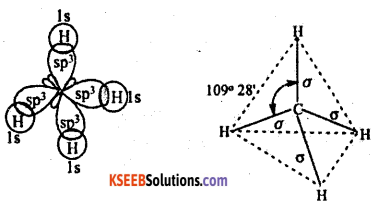The valence orbital contains impaired electron. Hence sp3 hybridized carbon combine with 4 hydrogen atom forms methane molecule.Question 5.
Explain sp2 hybridization in ethane molecule. C2H4 – ethene.
The molecular formula of ethene is C2H4
Electronic configuration of C is ground state – 1s2 2s2 2p2
Electronic configuration of C is excited state – 1s22s12p3
Valence orbital representation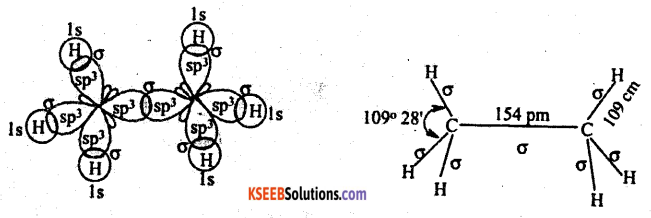sp2 hybridization involves 2 carbon atoms combines with 4 atoms of hydrogen.
In ethane molecule, there is 5 sigma and 1 pi bond. The angle of sp2 hybridized ethane is 120°.

Question 6.
Explain sp2 hybridization BF3 molecule.
BF3 molecule
Electronic configuration of B – 1s2 2s2 3P1
Valance orbital representation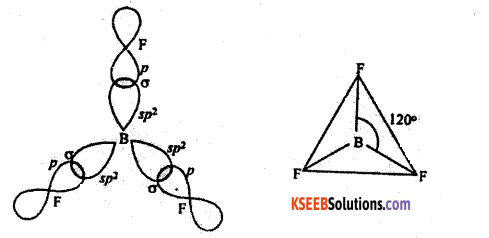Electronic configuration of F – 1s2 2s2 2P5
Valance orbital representation
Only one unpaired electrons is present in fluorine. Hence only 2pz orbital of fluorine involved in bond formation.
Structure – triangular planar.

Question 7.
Explain sp hybridisation with an example.
C2H2 – ethyne or acetylene
Electronic configuration of C is ground state -1s2 2s2 2P2
Electronic configuration of C excited state – 1s2 2s2 2P3
Valance orbital representation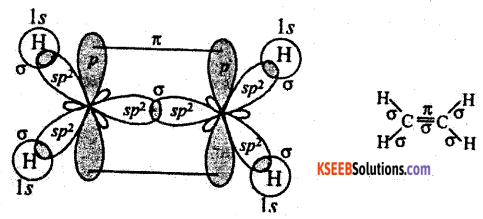Sp hybridised, C contains 2 impaired electron and one each in py and pz two sp hybridised C combines with two 1s1 orbital of H.
Two more hybrid orbitals each carbon overlap with Is atomic orbitals of hydrogen to form sigma bonds. The unhybridised 2pz orbitals of both the carbon atoms now overlap sideways to form carbon-carbon pi-bond.

Question 8.
Explain valence Shell Electron Pair Repulsion (VSEPR) theory.
Ans:
Bonding s The bond formation takes place if there exists impaired electron in the valance shell.

• Shape : The geometry or the shape of a molecule depends on the number of valence shell electrons surroundings the central atom.
• Repulsion : Electron pair tend to repell one another because electron clouds have similar charge.
Stability s As a result of electron pair repulsion these electron pairs try to stay apart as possible in order to attain minimum energy and maximum stability.
• Repulsive interaction : Lane pair with lane pair electrons are having maximum repulsive interaction, bond pair – bond pair electrons are having minimum repulsive interaction.
• When angle of repulsion decreases angle between the electron pair increases.
(109°. 28′ less repulsion, 104° more repulsion)Question 9.
Based on VSEPR theory explain the structure of ammonia.
Ammonia (NH3)
Electronic configuration of N : 1s2 2s2 2p3
Valance orbital representation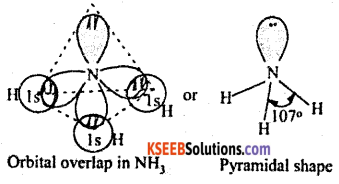1. When 2s2 orbital hybridised with 2px, 2py and 2pz forms the following sp3 hybridised structure..
2. In 2s2 orbital, the electrons are paired up hence they are not involved in bond formation called loan paired of electron (L.P).
3. When sp3 hybridized N combines with 3 hydrogen atom, forms pyramidal structure of ammonia.
Pyramidal structure angle = 107°

Question 10.
Based on VSEPR theory explain the structure of water.
Electronic configuration of O: 1s22s22p4
Valence orbital representation: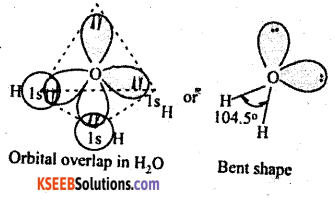1. When 2s2 orbital hybridised with 2sx, 2py and 2pz forms the following sp3 hybridisation structure.
2. In 2s2 and 2px2 , the electrons are paired up hence they are not involved in bond formation called loan paired of electron (L.P.)
3. When sp3 hybridised O combines with 2 hydrogen atom forms Bond structure water.

Question 11.
What are polar and Non Polar covalent bond explain with example.
Polar covalent molecules : In diatomic covalent molecule, the shared pair of electrons is displaced towards more electronegative atom present in the molecule is called polar covalent molecule. Example : HC1
Chlorine pulls the shared electron from hydrogen towards itself. Hence it is polar molecule.
H2O
Oxygen pulls the shared pair of electron from two hydrogen atom surrounding it. Hence it is a polar molecule.
Non Pollar covalent bond : In diatomic covalent molecule, the shared pair of electron is equally distributed between the atoms in the molecule.
Example: H2, O2, N2, O3 etc.Question 12.
Define dipole moment ? Comment on structure & dipole moment of CO2, BF3
It is the product of electric charge and distance between the positive and negative species present in the molecule.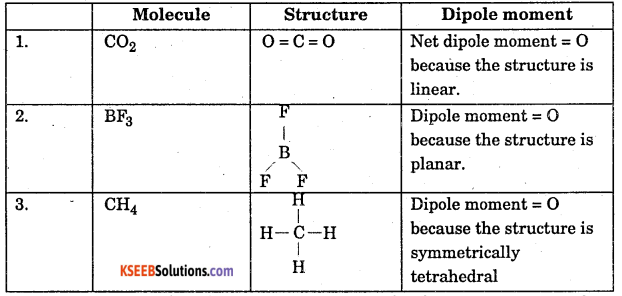The combining atom should attain octect structure by sharing one or more electron.

Question 13.
Define Hydrogen Bond ? Explain its type with example.
Electrostatic force of attraction that exists between hydrogen atom of one molecule and electronegative atom of same or other molecule is called hydrogen bond.
Type of hydrogen bond :

1. Intermolelcular hydrogen bond.
2. Intramolecular hydrogen bond.

1. Intermolecular hydrogen bond : Electrostatic force of attraction that exists between hydrogen atom of one molecule and electronegative atom of another molecule is called intermolecular hydrogen atom.
1. H2O .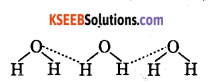2. Intramolecular Hydrogen bond : Electrostatic force of attraction that exists between hydrogen atom of one molecule electronegative atom of the same molecule. Example : Salicylic acid C6H4COOH(OH)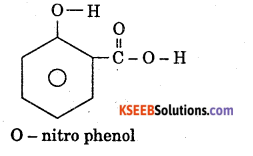### 1st PUC Chemistry Chemical Bonding and Molecular Structure Four / Five Marks Questions and Answers

Question 1.
Explain Valence Bond Theory.

• The covalent bond is formed by overlapping of those orbital which contains impaired electrons.
• When the overlapping is along axis, the overlapping becomes maximum and strong, called as o sigma bond.
• During overlapping, the electron present in the valance orbit must have opposite spin.
• Covalent molecule have lower energy than combining atoms. Therefore resulting molecule are always stable.
The extent of overlapping is ss > s-p > p-p.

Question 2.
Salient features of Molecular Orbital Theory (MOT). ‘